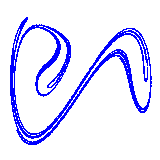Duffing Equation AnimationHere we treat a pendulum called Duffing equation. It is described by a differential equation written as d2x/dt2 = - δ dx/dt + x- x3 + γ cos(ω t) where δ=0.20, γ=0.30, and ω=1.0. Solving this equation numerically, a path x(t) is derived. Sampling it every T (s), we can get two-dimensional discrete-time data (x(nT), dx(nT)/dt) (n=0,1,...). If we choose the period of external force (2π/ω) as sampling time T, we can get the strange attractor. If we change the time when the sampling start, the structure of the attractor varies. The above animation is a collection of such sequences of attractors. The shape of attractor is changing with the same period as external force. You can observe the dynamics of the Duffing equation also in "attractor of Duffing equation" simulator.

The images used to make this animation are as follows:
0 / 1 / 2 / 3 / 4 / 5 / 6 / 7

<< Periodically Forced Pendulum 2 Animation / Lorenz Attractor Animation >>

Introduction to Chaos and Nonlinear Dynamics